# HSSlive: Plus One & Plus Two Notes & Solutions for Kerala State Board

## BSEB Class 10 Maths Chapter 3 Pair of Linear Equations in Two Variables Ex 3.5 Textbook Solutions PDF: Download Bihar Board STD 10th Maths Chapter 3 Pair of Linear Equations in Two Variables Ex 3.5 Book AnswersBSEB Class 10 Maths Chapter 3 Pair of Linear Equations in Two Variables Ex 3.5 Textbook Solutions PDF: Download Bihar Board STD 10th Maths Chapter 3 Pair of Linear Equations in Two Variables Ex 3.5 Book Answers

BSEB Class 10th Maths Chapter 3 Pair of Linear Equations in Two Variables Ex 3.5 Textbooks Solutions and answers for students are now available in pdf format. Bihar Board Class 10th Maths Chapter 3 Pair of Linear Equations in Two Variables Ex 3.5 Book answers and solutions are one of the most important study materials for any student. The Bihar Board Class 10th Maths Chapter 3 Pair of Linear Equations in Two Variables Ex 3.5 books are published by the Bihar Board Publishers. These Bihar Board Class 10th Maths Chapter 3 Pair of Linear Equations in Two Variables Ex 3.5 textbooks are prepared by a group of expert faculty members. Students can download these BSEB STD 10th Maths Chapter 3 Pair of Linear Equations in Two Variables Ex 3.5 book solutions pdf online from this page.

## Bihar Board Class 10th Maths Chapter 3 Pair of Linear Equations in Two Variables Ex 3.5 Books Solutions

 Board BSEB Materials Textbook Solutions/Guide Format DOC/PDF Class 10th Subject Maths Chapter 3 Pair of Linear Equations in Two Variables Ex 3.5 Chapters All Provider Hsslive

## How to download Bihar Board Class 10th Maths Chapter 3 Pair of Linear Equations in Two Variables Ex 3.5 Textbook Solutions Answers PDF Online?

2. Click on the Bihar Board Class 10th Maths Chapter 3 Pair of Linear Equations in Two Variables Ex 3.5 Answers.
3. Look for your Bihar Board STD 10th Maths Chapter 3 Pair of Linear Equations in Two Variables Ex 3.5 Textbooks PDF.
4. Now download or read the Bihar Board Class 10th Maths Chapter 3 Pair of Linear Equations in Two Variables Ex 3.5 Textbook Solutions for PDF Free.

## BSEB Class 10th Maths Chapter 3 Pair of Linear Equations in Two Variables Ex 3.5 Textbooks Solutions with Answer PDF Download

Find below the list of all BSEB Class 10th Maths Chapter 3 Pair of Linear Equations in Two Variables Ex 3.5 Textbook Solutions for PDF’s for you to download and prepare for the upcoming exams:

## BSEB Bihar Board Class 10th Maths Solutions Chapter 3 Pair of Linear Equations in Two Variables Ex 3.5

Question 1.
Which of the following pairs of linear equations has unique solution, no solution, or infinitely many solutions? In case there is a unique solution, find it by using cross multiplication method.
1. x – 3y – 3 = 0
3x – 9y – 2 = 0

2. 2x + y = 5
3x + 2y = 8

3. 3x – 5y = 20
6x – 10y = 40

4. x – 3y – 7 = 0
3x – 3y – 15 = 0
Solution:
1. The given system of equations is
x – 3y – 3 = 0 and
3x – 9y – 2 = 0
These are of the form
a1x + b1y + c1 = 0
and a2x + b2y + c2 = 0
where a1 = 1, b1 = – 3, c1 = – 3 and a2 = 3, b2 = – 9, c2 = – 2So, the given system of equations has no solution, i.e., it is inconsistent.

2. The given system of equations may be written as 2x + y – 5 = 0 and 3x + 2y – 8 = 0
These are of the form
a1x + b1y + c1 = 0 and x2x + b2y + c2 = 0
where a1 = 2, b1 = 1, c1 = – 5 and a2 = 3, b2 = 2 and c2 = – 8So, the given system of equations has a unique solution.
To find the solution, we use the cross-multiplication method. By cross-multiplication, we have: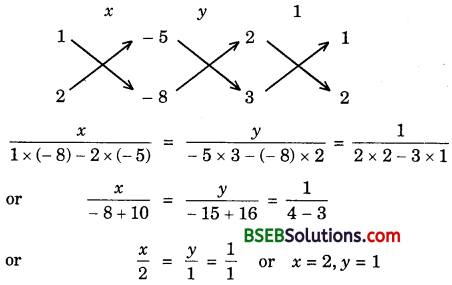Hence, the given system of equations has a unique solution given by x = 2, y = 1.

3. The given system of equations may be written as
3x – 5y – 20 – 0 and 6x – 10y – 40 = 0
The given equations are of the form
a1x + b1y + c1 = – 20 and a2 = 6, b2 = – 10, c2 = – 40.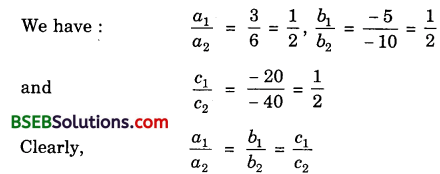So, the given system of equations has infinitely many solutions.

4. The given system of equations is
x – 3y – 7 = 0 and 3x – 3y – 15 = 0
The given equations are of the form
a1x + b1y + c1 = 0 and a2x + b2y + c2 = 0
where a1 = 1, b1 = – 3, c1 = – 7 and a2 = 3, b2 = – 3, c2 = – 15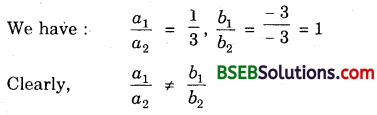So, the given system of equations has a unique solution.
To find the solution, we use cross-multiplication method. By cross-multiplication, we have: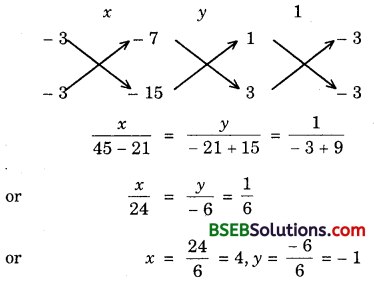Hence, the given system of equations has a unique solution given by
x = 4, y = – 1

Question 2.
1. For which values of a and b does the following pair of linear equations have an infinite number of solutions?
2x + 3y = 7
(a – b)x + (a + b)y = 3a + b – 2
2. For which value of k will the following pair of linear equations have no solution?
3x + y = 1
(2k – 1)x + (k – 1)y = 2k + 1
Solution:
1. We know that the system of equations
a1x + b1y + c1 = 0 and a2x + b2y + c2 = 0 has infinite number of solutions if
𝑎1𝑎2=𝑏1𝑏2=𝑐1𝑐2
∴ The given system of equations can be written as follows:
2x + 3y – 7 = 0
(a – b)x + (a – t – b)y – 3a – b + 2 = 0
It will have infinite number of solutions, if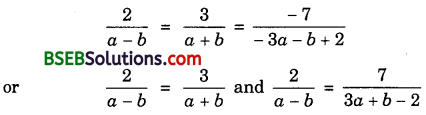2a + 2b = 3a – 3b and 6a + 2b – 4 = 7a – 7b
a = 5b and a – 9b = – 4
Putting a = 5b in a = 5b = – 4, we get
56 – 96 = – 4 or – 46 = – 4 or 6 = 1
Putting b = 1 in a = 56, we get
a = 5(1) = 5
Hence, the given system of equations will have infinitely many solutions, if
a = 5 and b = 1

2. We know that the system of equations
a1x + b1y + c1 = 0 and a2x + b2y + c2 = 0
has no solution if 𝑎1𝑎2=𝑏1𝑏2≠𝑐1𝑐2
The given system of equations can be written as follows :
3x + y – 1 = 0
(2k – 1)x + (k – 1)y – 2k – 1 = 0
It will have no solution, if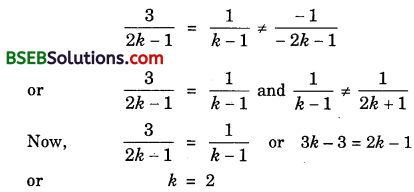Clearly, for k = 2, we have:
1𝑘−1 ≠ 12𝑘+1
Hence, the given system of equations will have no solution, if k = 2.

Question 3.
Solve the following pair of linear equations by the substitution and cross-multiplication methods:
8x + 5y = 9
3x + 2y = 4
Solution:
The given system of equations is
8x + 5y = 9 …………….. (1)
and 3x + 2y = 4 ……………. (2)
By substitution method:
Substituting y = 9−8𝑥5 in (2), we get
or 15x + 18 – 16x = 20 or – x = 2 or x = – 2
Putting x = – 2 in (1), we get
8(- 2) + 5y = 9 or 5y = 9 + 16 = 25
or y = 255 = 5
Hence, x = – 2, y = 5 is the solution of the given system of equations.

By cross-multiplication method:
The given equations can be written as follows:
8x + 5y – 9 – 0
3x + 2y – 4 = 0
So, we have:Hence, x = – 2, y = 5 is the solution of the given system of equations.

Question 4.
Form the pair of linear equations in the following problems and find their solutions (if they exist) by any algebraic method:
1. A part of monthly hostel charges is fixed and the remaining depends on the number of days one has taken food in the mess. When a student A takes food for 20 days, she has to pay Rs 1000 as hostel charges, whereas a student B, who takes food for 26 days, pays Rs 1180 as hostel charges. Find the fixed charges and the cost of food per day.

2. A fraction becomes 13 when 1 is subtracted from the numerator and it becomes 14 when 8 is added to its denominator. Find the fraction.

3. Yash scored 40 marks in a test, getting 3 marks for each right answer and losing 1 mark for each wrong answer. Had 4 marks been awarded for each correct answer and 2 marks been deducted for each incorrect answer, then Yash would have scored 50 marks. How many questions were there in the test?

4. Places A and B are 100 km apart on a highway. One car starts from A and another from B at the same time. If the cars travel in the same direction at different speeds, they meet in 5 hours. If they travel towards each other, they meet in 1 hour. What are the speeds of the two cars?

5. The area of a rectangle gets reduced by 9 square units, if its length is reduced by 5 units and breadth is increased by 3 units. If we increase the length by 3 units and the breadth by 2 units, the area increases by 67 square units. Find the dimensions of the rectangle.
Solution:
1. Let the fixed charges be Rs x and charges per day be Rs y.
∴ By the given conditions,
x + 20y = 1000 …………….. (1)
and x + 26y = 1180 ……………….. (2)
Subtracting (1) from (2), we get
6y = 180 or y = 30
From (1), x + 20 × 30 = 1000
x = 1000 – 600 = 400
∴ Fixed charges = Rs 400
and cost of food per day = Rs 30

2. Let the fraction be 𝑥𝑦.
According to the given conditions:
𝑥−1𝑦 = 13 or 3x – 3 = y
or 3x – y – 3 = 0 …………….. (1)
and 𝑥𝑦+8 = 14 or 4x = y + 8
or 4x – y – 8 = 0 ………………. (2)
Solving (1) and (2) by cross-multiplication method, we have:Hence, the required fraction is 512.

3. Let Yash answer x questions correctly and y questions incorrectly. According to the given conditions:
3x – y = 40 ………………. (1)
and 4x – 2y = 50 ………………….. (2)
Multiplying (1) by 2 and subtracting (2) from the result so obtained, we get
2x = 30 or x = 15
From (1), 3 × 15 – y = 40 or – y = 40 – 45
or – y = – 5 or y = 5
∴ Total number of questions in the test are 15 + 5 = 20.

4. Let X and Y be two cars starting from places A and B respectively. Let the speed of car X be x km/h and that of Y be y km/h.
Case I: When two cars move in the same direction.
Let these cars meet at point Q. Then,
Distance travelled by car X = AQ
Distance travelled by car Y = BQ
It is given that two cars meet in 5 hours.
∴ Distance travelled by car X in 5 hours = 5x km
or AQ = 5x
Distance travelled by car Y in 5 hours = 5y km
or BQ = 5y
Clearly, AQ – BQ = AB
or 5x – 5y = 100
or x – y = 20 [∵ AB = 100 km]Case II: When two cars move in opposite directions.
Let these cars meet at point P. Then,
Distance travelled by car X = AP
Distance travelled by car Y = BP
In this case, two cars meet in 1 hour.
∴ Distance travelled by car X in 1 hour = x km
or AP = x
Distance travelled by car Y in 1 hour = y km
or BP = y
Clearly, AP + PB = AB
or x + y = 100 ……………. (2) [∵ AB = 100 km]
Solving (1) and (2), we have:
x = 60, y = 40
Hence, speed of car X is 60 km/h and speed of car Y is 40 km/h.

5. Let the length and breadth of the rectangle be x and y units respectively. Then,
Area = xy sq. units
If length is reduced by 5 units and breadth is increased by 3 units, then area is reduced by 9 sq. units.
∴ xy – 9 = (x – 5)(y + 3)
or xy – 9 = xy + 3x – 5y – 15
or 3x – 5y – 6 = 0 …………….. (1)
When length is increased by 3 units and breadth by 2 units, the area is increased by 67 sq. units.
∴ xy + 67 = (x + 3 )(y + 2)
or xy + 67 = xy + 2x + 3y + 6
or 2x + 3y – 61 = 0 ………………… (2)
Solving (1) and (2), we get
𝑥305+18 = −𝑦−183+12 = 19+10
or x = 32319 = 17, y = 17119 = 9
Hence, the length and breadth of the rectangle are 17 units and 9 units respectively.

## Bihar Board Class 10th Maths Chapter 3 Pair of Linear Equations in Two Variables Ex 3.5 Textbooks for Exam Preparations

Bihar Board Class 10th Maths Chapter 3 Pair of Linear Equations in Two Variables Ex 3.5 Textbook Solutions can be of great help in your Bihar Board Class 10th Maths Chapter 3 Pair of Linear Equations in Two Variables Ex 3.5 exam preparation. The BSEB STD 10th Maths Chapter 3 Pair of Linear Equations in Two Variables Ex 3.5 Textbooks study material, used with the English medium textbooks, can help you complete the entire Class 10th Maths Chapter 3 Pair of Linear Equations in Two Variables Ex 3.5 Books State Board syllabus with maximum efficiency.

## FAQs Regarding Bihar Board Class 10th Maths Chapter 3 Pair of Linear Equations in Two Variables Ex 3.5 Textbook Solutions

#### How to get BSEB Class 10th Maths Chapter 3 Pair of Linear Equations in Two Variables Ex 3.5 Textbook Answers??

Students can download the Bihar Board Class 10 Maths Chapter 3 Pair of Linear Equations in Two Variables Ex 3.5 Answers PDF from the links provided above.

#### Can we get a Bihar Board Book PDF for all Classes?

Yes you can get Bihar Board Text Book PDF for all classes using the links provided in the above article.

## Important Terms

Bihar Board Class 10th Maths Chapter 3 Pair of Linear Equations in Two Variables Ex 3.5, BSEB Class 10th Maths Chapter 3 Pair of Linear Equations in Two Variables Ex 3.5 Textbooks, Bihar Board Class 10th Maths Chapter 3 Pair of Linear Equations in Two Variables Ex 3.5, Bihar Board Class 10th Maths Chapter 3 Pair of Linear Equations in Two Variables Ex 3.5 Textbook solutions, BSEB Class 10th Maths Chapter 3 Pair of Linear Equations in Two Variables Ex 3.5 Textbooks Solutions, Bihar Board STD 10th Maths Chapter 3 Pair of Linear Equations in Two Variables Ex 3.5, BSEB STD 10th Maths Chapter 3 Pair of Linear Equations in Two Variables Ex 3.5 Textbooks, Bihar Board STD 10th Maths Chapter 3 Pair of Linear Equations in Two Variables Ex 3.5, Bihar Board STD 10th Maths Chapter 3 Pair of Linear Equations in Two Variables Ex 3.5 Textbook solutions, BSEB STD 10th Maths Chapter 3 Pair of Linear Equations in Two Variables Ex 3.5 Textbooks Solutions,
Share: